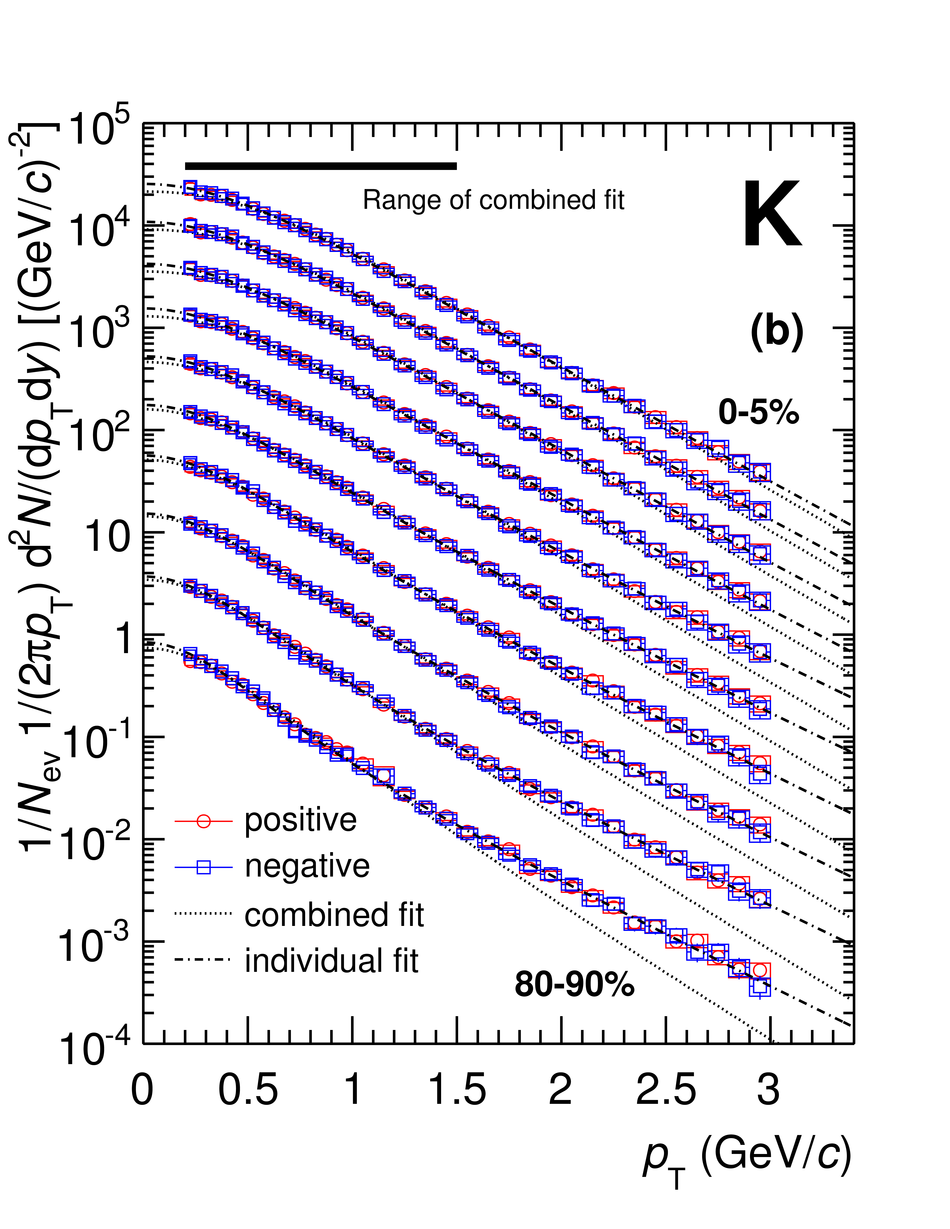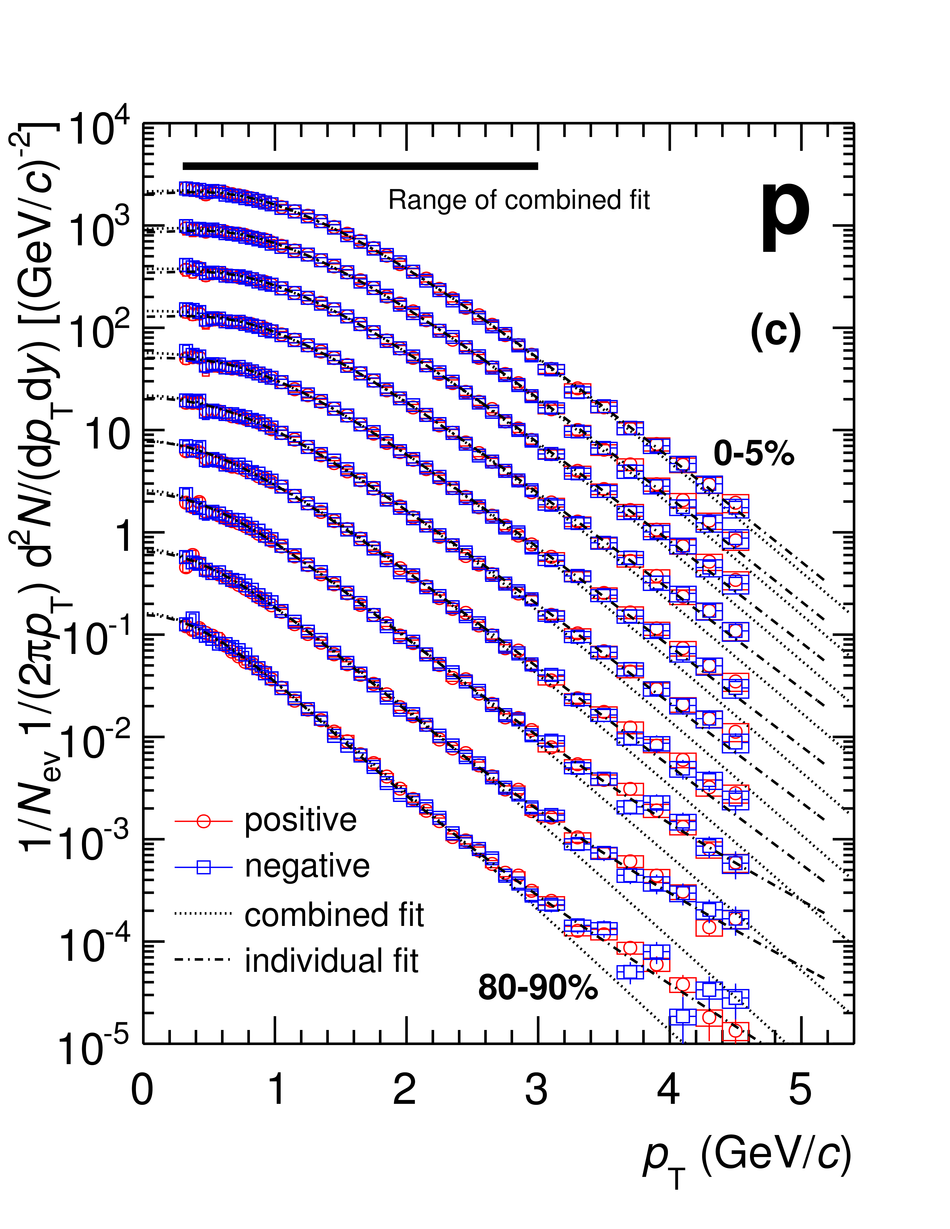# Figure 4

 Transverse momentum ($\pt$) distribution of (a) $\pi$, (b) K, and (c) p as a function of centrality, for positive (circles) and negative (squares) hadrons. Each panel shows central to peripheral data; spectra scaled by factors $2^n$ (peripheral data not scaled). Dashed curves: blast-wave fits to individual particles; dotted curves: combined blast-wave fits (see text for details). Statistical (error bars) and systematic (boxes) uncertainties plotted. An additional normalization uncertainty (see table 3) has to be added in quadrature.